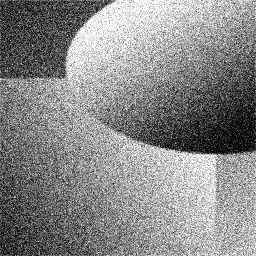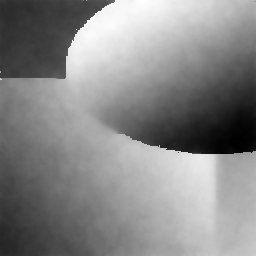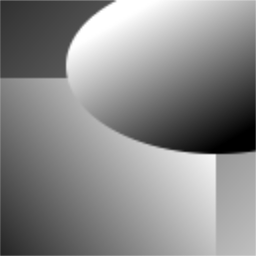DGtalTools  1.3.beta
imageProcessing/at-u0-v1

Computes a piecewise smooth approximation of a grey-level or color image, by optimizing the Ambrosio-Tortorelli functional (with u a 0-form and v a 1-form).

Author(s) of this documentation: Marion Foare, Jacques-Olivier Lachaud

Usage: at-u0-v1 -i [input.pgm]

(for grey-level image restoration)

Usage: at-u0-v1 -i [input.ppm]

(for color image restoration)

The Ambrosio-Tortorelli functional is a classical relaxation of the Mumford-Shah functional.

Given an input grayscale image, defined in an open bounded domain $$\Omega$$, we represent its gray levels by a function $$g \in L^{\infty}(\Omega)$$. In the Ambrosio-Tortorelli functional , one wants to find a function $$u \in SBV(\Omega)$$ which is a smooth approximation of the input image $$g$$. The Ambrosio-Tortorelli functional  is defined by

$\displaystyle AT_{\varepsilon}(u,v) = \int_\Omega \alpha |u-g|^2 + v^2 |\nabla u|^2 + \lambda \varepsilon |\nabla v|^2 + \frac{\lambda}{4 \varepsilon} |1-v|^2 dx,$

for functions $$u,v \in W^{1,2}(\Omega)$$ with $$0 \leq v \leq 1$$.

In AT functional, function $$v$$ is a smooth approximation of the set of discontinuities, and takes value close to 0 in this set, while being close to 1 outside discontinuities. A remarkable property of this functional is that it $$\Gamma$$-converges to (a relaxation of) MS functional as $$\varepsilon$$ tends to 0 (see ). The intuition is that a large $$\varepsilon$$ induces a solution with a fuzzy set of discontinuities, which is then progressively narrowed to the crisp 1-dimensional set of discontinuites as $$\varepsilon$$ goes to 0.

We discretize AT with discrete calculus and define $$u$$ and $$g$$ on the vertices and $$v$$ on the edges. We denote this formulation AT10. Gray levels are seen as point mass on the center of pixels, so that functions $$u$$ and $$g$$ are both 0-forms, while $$v$$ is a dual 1-form in between $$u$$. It follows:

$\displaystyle AT10(u,v) = \Sigma_{i=1}^n \alpha \langle u_i - g_i , u_i - g_i \rangle_0 + \langle v , \mathbf{d_0} u_i \rangle_1 \langle v , \mathbf{d_0} u_i \rangle_1 \\ + \lambda \varepsilon \langle (\mathbf{d_1} + \bar{\mathbf{\star}} \bar{\mathbf{d_1}} \mathbf{\star}) v , (\mathbf{d_1} + \bar{\mathbf{\star}} \bar{\mathbf{d_1}} \mathbf{\star}) v \rangle_1 + \frac{\lambda}{4\varepsilon} \langle 1 - v , 1 - v \rangle_1.$

For more details, see Image restoration and inpainting with Ambrosio-Tortorelli functional

Allowed options are:

Positionals:
1 TEXT:FILE REQUIRED the input image PPM filename.
Options:
-h,--help Print this help message and exit
-i,--input TEXT:FILE REQUIRED the input image PPM filename.
-m,--inpainting-mask TEXT the input inpainting mask filename.
-o,--output TEXT=AT the output image basename.
-l,--lambda FLOAT the parameter lambda.
-1,--lambda-1 FLOAT=0.3125 the initial parameter lambda (l1).
-2,--lambda-2 FLOAT=0.0005 the final parameter lambda (l2).
-q,--lambda-ratio FLOAT=1.41421 the division ratio for lambda from l1 to l2.
-a,--alpha FLOAT=1 the parameter alpha.
-e,--epsilon the initial and final parameter epsilon of AT functional at the same time.
--epsilon-1 FLOAT=2 the initial parameter epsilon.
--epsilon-2 FLOAT=0.25 the final parameter epsilon.
--epsilon-r FLOAT=2 sets the ratio between two consecutive epsilon values of AT functional.
-n,--nbiter INT=10 the maximum number of iterations.
--image-snr TEXT the input image without deterioration if you wish to compute the SNR.
-p,--pixel-size INT=1 the pixel size for outputing images (useful when one wants to see the discontinuities v on top of u).
-c,--color-v TEXT=0xff0000 the color chosen for displaying the singularities v (e.g. red is 0xff0000).
-v,--verbose INT=0 the verbose level (0: silent, 1: less silent, etc).

example:

./imageProcessing/at-u0-v1 -i ../imageProcessing/Images/degrade-b04.pgm --image-snr ../imageProcessing/Images/degrade.pgm -a 0.05 --epsilon-1 4 --epsilon-2 0.25 -l 0.005 -p 2 -c 0xff0000 -o degrade
 Input image g Reconstructed image u Perfect imageInput image (noise = 0.4)AT01 alpha=0.05 lambda=0.005Perfect image SNR of g = 21.9183 SNR of u = 34.4426 Perfect image
Note
Other restoration examples, parameter analysis, and image inpainting examples may be found in Image restoration and inpainting with Ambrosio-Tortorelli functional.

 Marion Foare, Jacques-Olivier Lachaud, and Hugues Talbot. "Image restoration and segmentation using the Ambrosio-Tortorelli functional and discrete calculus." In Proceedings of the IAPR International Conference on Pattern Recognition (ICPR2016), Cancun, Mexico, 2016.

 Marion Foare, Jacques-Olivier Lachaud, and Hugues Talbot. "Numerical implementation of the Ambrosio-Tortorelli functional using discrete calculus and application to image restoration and inpainting." In Proceedings of 1st Workshop on Reproducible Research In Pattern Recognition (RRPR 2016), Springer LNCS. To appear.

 Matteo Focardi. "On the variational approximation of free- discontinuity problems in the vectorial case." Mathematical Models and Methods in Applied Sciences 11.04 (2001): 663-684.